# Quantum Mechanics

## 2. A First Approach to Classical Mechanics

### 2.5 Poisson Brackets and Hamiltonian Mechanics

Let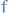and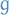be two smooth functions on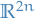, where an element ofis thought of as a pair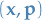, with

•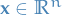representing position of a particle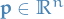representing the momentum of a particle

Then the Poisson bracket ofand, denoted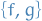is the function ongiven by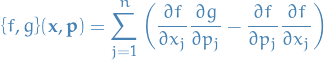For all smooth functions,and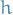onwe have the following:

1.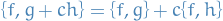for all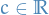2.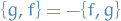3.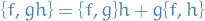4. Jacobi identity: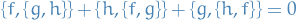The position and momentum functions satisfy the following Poisson bracket relations: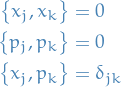If a particle in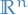has the usual sort of energy function (kinetic energy plus potential energy), we have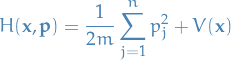Wit the Hamiltonian, and as usual, having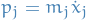, we can write Netwon's laws as: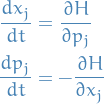These equations we refer to has Hamilton's equations.

If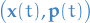is a solution of the Hamilton's equation, then for any functionon, we have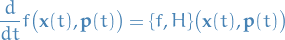Call a smooth functionona conserved quantity if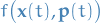is independent of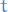for each solutionof Hamilton's equations.

Thenis a conserved quantity if and only if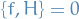In particular, the Hamiltonian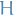is a conserved quantity.

Solving Hamilton's equatons ongives rise to a flow on, that is, a family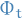of diffeomorphisms of, where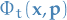is equal to the solution at timeof Hamilton's equations with initial conditions.

Since it is possible (depending on the choice of potential function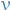) that a particle can escape to infinity in finite time, the mapsare not necessarily defined on all of, but only on some subset therof.

Ifis defined on all ofwe say it's complete.

The flow associated with Hamilton's equations, for an arbitrary Hamitonian function, preserves the (2n)-dimensional volume measure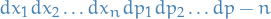What this means, more precisely, is that if a measurable set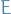is contained in the domain offor some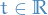, then the volume of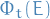is equal to the volume of.